# The Fascinating Plimpton 322

Mathematics is one of the most powerful tools in human existence. From basic counting and grocery shopping to advanced applications such as in computing and aerospace, math plays important roles and can be found everywhere. However, the math system was not built in one day. All the current have their past. Believe it or not, some archaeological findings in mathematics are still fascinating to us today, even though they were from hundreds or even thousands years ago. The clay tablet named Plimpton 322 is one of these valuable and interesting findings (Fig. 1).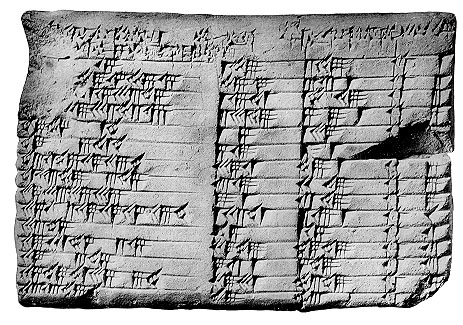Figure 1. The clay tablet Plimpton 322.

Plimpton 322 is a great presentation of how advanced Babylonian mathematics was. The clay tablet was written around 1800 BC. The math represented on the table is now known as Pythagorean triples. It was long before the development of Greek mathematics. The clay tablet was purchased by George Arthur Plimpton from an archaeological dealer in 1923. It now lies in the G.A Plipton Collection at Columbia University numbered 322. This is also how the tablet was named Plimpton 322.

The table on the tablet was inscribed with four columns and fifteen rows. In the rightmost column, which is the 4th column, it includes the number of rows which is numbered from 1 to 15. Before we jump into the math, we need to know how numbers were recorded and how to translate these symbols to the math we understand. In the table, 1 is symbolized as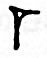which also called a “stroke”. From 2 to 9, the numbers were inscribed by combining multiple of strokes. Figure 2 shows how numbers 2 through 9 were written in the table. The number 10 was written as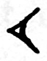.The same combination fashion works for numbers 10, 20, 30, 40, and 50 (Fig.3). How to write numbers between 10 and 20 or 20 and 30? the number system on the tablet still relys on combining “strokes”. 11 was written aswhich is an addition or combination result of 1 and 10. 0 is recorded as blank space. At this moment, you might think it is the same system as the system similar to the one we use today. However it is not quite. The math system we use now is based on 10. However, the system the Babylonian used was based on 60. It means that if the number is written as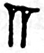, which tansclates to 1;2;1. It mathmatically represents 1*(60*60)+2*(60)+1=3721.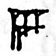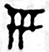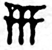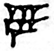Figure 2. How the numbers from 2 to 9 were inscribed on Plimpton 322.Figure 3. How the numbers 10, 20, 30, 40 were inscribed on Plimpton 322.

With the basic translation we just learned, now we can translate those symbols to the numbers we understand for the 2nd, 3rd and 4th columns. For the 4th column, it is listed 1 to 15 for each rows. The numbers here represent the row numbers (Fig. 4). Now we can also understand the 2nd column by using the addition base 60 number system (Fig. 5). You can go back to the earlier mathematical example of calculating 1;2;1 if you have any difficulties. When we have the basic understanding of how numbers are inscribed, we now need to know what these numbers mean or how the math works on the tablet. Archeologists translated the names on the top of each column which offers more information to the later discovery. On the 2nd column it has the word “width” and the 3rd it says “diagonal”. Mathematicians thought it might relate to Pythagorean triples. The results showed the guess was right if you ignore the error the tablet maker had (the corrected numbers are marked as red in Figure 5). For each row, the diagonal d, width w, and the unknown length of the last side of triangle l, make an equation w2+l2=d2. It is also true that all the ls are integers. So now the question remains on what does the 1st column do? It is actually the fraction of d^2/l^2. From Figure 1, every single row in the 1st column consists a series of numbers. The numbers can be translated into decimal numbers. For instance, in the first row where d=169 and l=120. Therefore, the fraction of d2/l2 should be about 1.9834. The first column has numbers 1;59;0;15. How is that 1.9834? On our normal 10 based system, for instance, 1.234 can be written as 1+2/10+3/(10*10)+ 4/(10*10*10). The same method also holds for the tablet decimal numbers. However, the only difference is that the numbers are 60 based. Therefore, 1:59:0:15, which is the decimal number inscribed on the fourth row of the 1st column can written as 1+59/(60)+0/(60*60)+15/(60*60*60)=1.9834.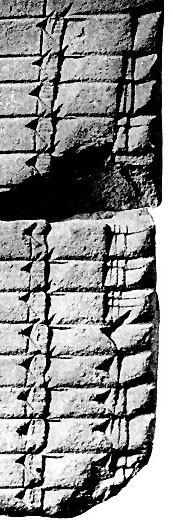Figure 4. The 4th column of Plimpton 322 (From the top to the bottom row, number of rows were inscribed which is 1 to 15).Figure 5. The 2nd and 3rd columns of Plimpton 322 (On each picture’s left side, the numbers on the left are the numbers decrypted from the inscribed symbols, the numbers in blue are the results after addition).

Who made Plimpton 322 and what was the purpose of making it? Eleanor Robson, a Plimpton 322 researcher, believes that it was made by a male due to geographical reason. All the female scribes from ancient Mesopotamia lived much further north to where this tablet was made. Robson also does not agree that the tablet author was a professional mathematician because the professional academic disciplines is a phenomenon of the very recent past. There are two possibilities to the author’s identity which Robson brought up. First is that the author could have been a trainee scribe or a teacher. He might have taught simple math techniques in scribal schools. He also knew the document format used by the temple and palace administrators of Larsa . Therefore, he was not a student. This leaves him highly likely to be a professional bureaucratic scribe. Robson also believes that the tablet served for teaching purposes. The tablet is similar to problem lists. This is likely to explain why the l numbers are missing on the tablet . They are all integers and waiting to be solved from the known values. The fifteen rows with the same method also indicate that the teacher might offer this tablet for students to practice repeatedly on those problems.

Now the math puzzle of the ancient tablet Plimpton 322 is finally solved. However, the exact answers for how the tablet was created or who was the author still remain elusive. It is fascinating for us to know how incredibly advanced Babylonian math was back into the time around 1800 BC. They knew Pythagorean triples which today is still super commonly used in math and its fields of application. We can clearly see that the history of mathematics is also a part the history of human existence. Mathematics was created, developed and is still developing and helping human beings to reach new and more advanced eras in human history.

Reference:
 Casselman, Bill. “The Babylonian Tablet Plimpton 322.” The University of British Columbia. Web. 8 Feb. 2015.
 Neugebauer, O.; Sachs, A. J. (1945), Mathematical Cuneiform Texts, American Oriental Series 29, New Haven: American Oriental Society and the American Schools of Oriental Research, pp. 38–41.
 Joyce, David. “PLIMPTON 322.” Clark University. Web. 8 Feb. 2015.
 Eleanor Robson. Words and Pictures: New Light on Plimpton 322, American Mathematics Monthly 109, 2002.